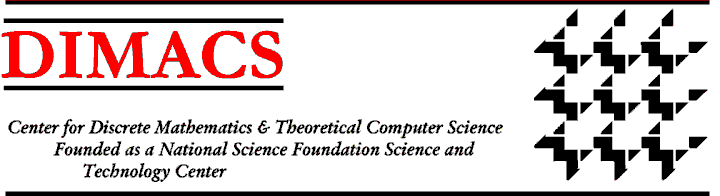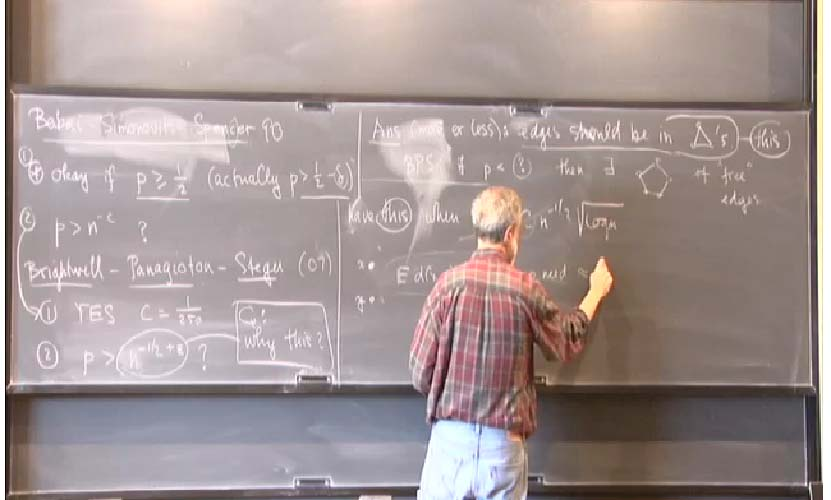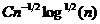Mantel’s Theorem for Random Graphs[May, 2012] Rutgers graduate student Bobby DeMarco and his advisor Jeffry Kahn (pictured) have determined when the size of the largest triangle-free subgraph and the largest bipartite subgraph of a random graph are likely to be equal. This is the “random graph version” of a classic (1907) result by Mantel showing that the sizes are equal in a complete graph.

If we let b(G) be the size of the largest bipartite subgraph of a graph G and t(G) be the size of the largest triangle-free subgraph, then t(G) is at least as large as b(G) because bipartite graphs are triangle free. An open question from about 20 years ago asked: for what p is it likely that t(G)=b(G) for a random graph G(n,p), where n is the number of vertices and p is the probably with which each edge appears? DeMarco and Kahn settled this question by showing that if p >for a suitable constant C, then with probability going to one as n goes to infinity, t(G)=b(G).  This result is tight up to the value of constant C.

For roughly the past two decades, demonstrating analogues of extremal results for random structures has been a very active area of research. DeMarco and Kahn’s result is one such analogue, but several celebrated extremal results also have natural analogues in a random setting.  For example, random analogues of Ramsey’s Theorem, Szemeredi’s Theorem on arithmetic progressions, and Turan’s Theorem on the density of graphs without a k-sized clique have all been demonstrated in the past twenty years.

This new result was part of DeMarco’s PhD dissertation on the mathematical structure of random graphs which he successfully defended in May 2012. DeMarco received a 3-year fellowship through DIMACS/CCICADA to support his graduate studies in mathematics. The fellowship was funded by a grant to DIMACS/CCICADA from the Department of Homeland Security.DIMACS Homepage
Contacting the Center